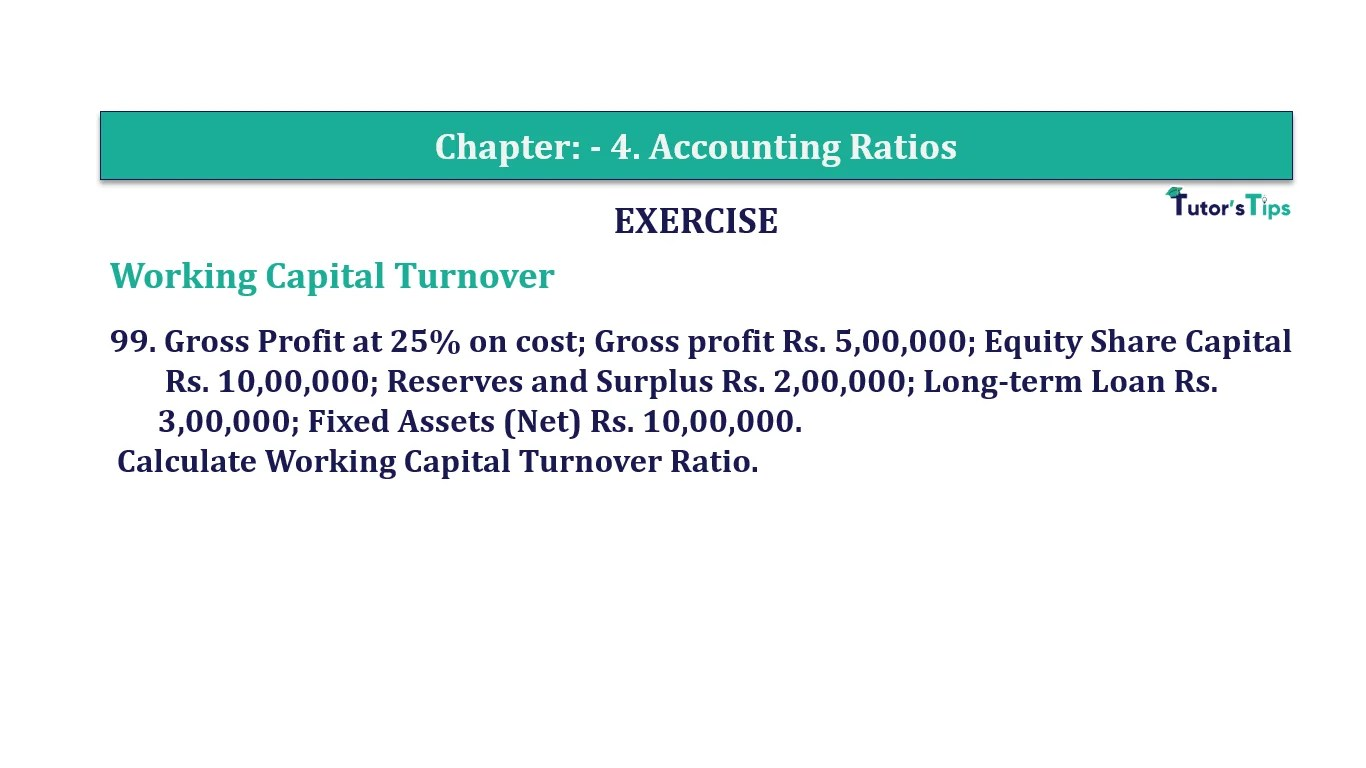# Question 99 Chapter 4 of +2-B – T.S. Grewal 12 ClassQuestion No. 99- Chapter No.4 - T.S. Grewal +2 Book Part B

Question 99 Chapter 4 of +2-B

Working Capital Turnover

99. Gross Profit at 25% on cost; Gross profit Rs. 5,00,000; Equity Share Capital Rs. 10,00,000; Reserves and Surplus Rs. 2,00,000; Long-term Loan Rs. 3,00,000; Fixed Assets (Net) Rs. 10,00,000.
Calculate Working Capital Turnover Ratio.

### The solution of Question 99 Chapter 4 of +2-B: –

 Gross Profit = 25% on Cost Cost of Goods Sold = X

 Gross Profit = X + 25 3
 Gross Profit = 25x 100
 Rs. 5,00,000 x 100 = 25x
 X = Rs.5,00,00,000 25 X = Rs. 20,00,000

 Net Sales = Cost of Goods Sold + Gross Profit = Rs. 20,00,000 + Rs. 5,00,000 = Rs. 25,00,000 Working Capital = Capital Employed – Fixed Assets = Rs. 15,00,000 – Rs. 10,00,000 = Rs. 5,00,000

 Working Capital Turnover Ratio = Cost of Goods Sold Working Capital
 = Rs. 25,00,000 Rs. 5,00,000 = 5 Times

Balance Sheet: Meaning, Format & Examples

Comment if you have any question.

Also, Check out the solved question of previous Chapters: –